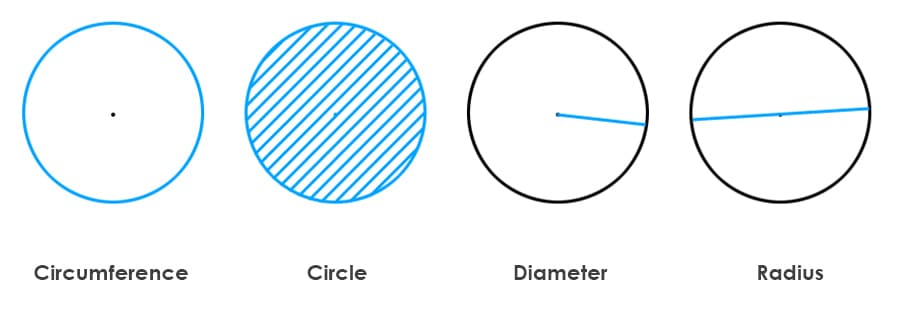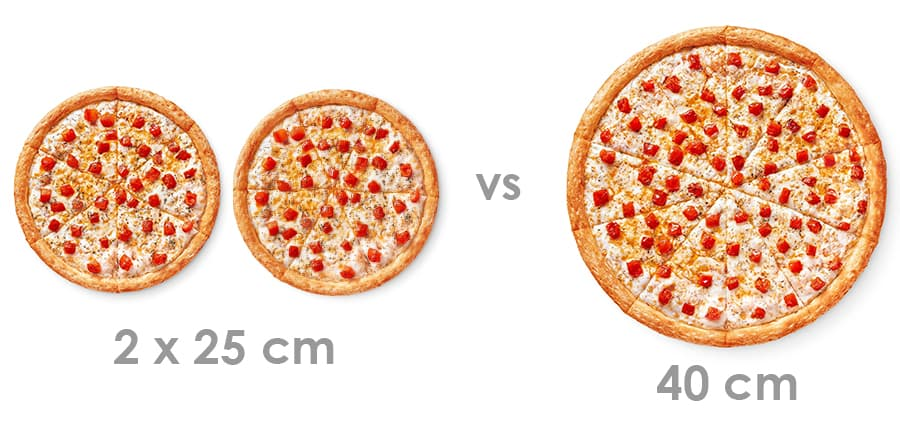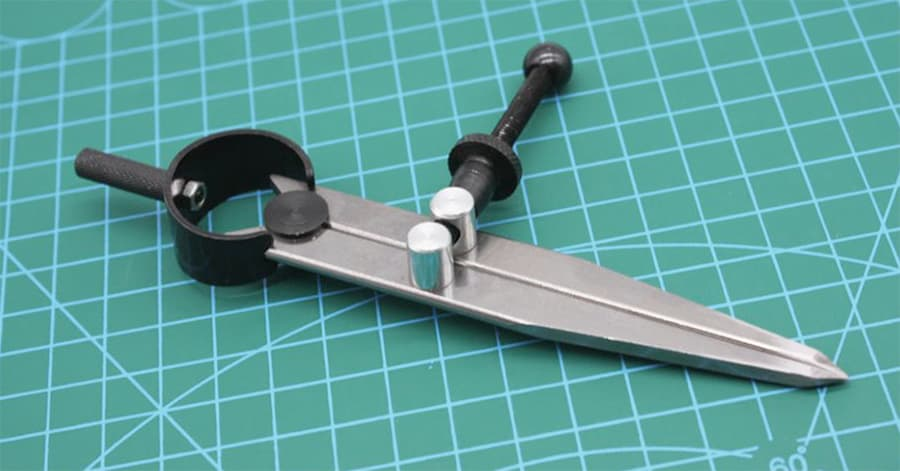# Circle Area Calculator

Calculate the area of a circle online based on the diameter, radius, or circumference.

Circle area

0copy

Contents

## What is Circle Area and How Calculate it?A circle is a two-dimensional shape that is defined by a set of points that are equidistant from a center point. The area of a circle is the measure of the surface enclosed by the circle. It is commonly measured in square units such as square meters (m²) or square feet (ft²).

The formulas for calculating the area of a circle is:

The formula to calculate the area of a circle using the radius is:

A = πr²

Where: A = Area of the circle π = A mathematical constant approximately equal to 3.14 (pi) r = The radius of the circle

To use this formula, simply square the radius of the circle and multiply it by π to obtain the area. For example, if the radius of a circle is 5 units, the area of the circle would be:

A = πr² = π(5²) = π(25) = 78.5 square units

So, the area of a circle with a radius of 5 units would be approximately 78.5 square units.

### Using Diameter

A = π * (diameter/2)²

where π (pi) is a mathematical constant approximately equal to 3.14159, and the diameter is the length of a line passing through the center of the circle and touching two points on its circumference.

For example, if the diameter of a circle is 10 cm, then the area of the circle would be:

Area = π * (10/2) 2 = π * 5² = 78.54 square centimeters (rounded to two decimal places)

### Using Circumference

A = (circumference²) / (4π)

where the circumference is the distance around the circle.

For example, if the circumference of a circle is 20 cm, then the area of the circle would be:

Area = (20²) / (4π) = 100 / π = 31.83 square centimeters (rounded to two decimal places)

## How to Use a Circle Area Calculator?

Using a circle area calculator is a simple and straightforward process. Follow these steps to calculate the area of a circle using a circle area calculator:

Step 1. Determine the radius, diameter or circumference of the circle. Measure the radius, diameter or circumference of the circle using a ruler or tape measure.

Step 2. Open the circle area calculator. There are many online circle area calculators available on the internet. Open the circle area calculator in your web browser.

Step 3. Enter the the parameters of the circle into the appropriate field on the calculator. Make sure you enter the value in the correct unit (e.g. centimeters, inches, meters).

Step 4. Press calculate. Once you have entered the parameters of the circle, click the "calculate" button on the calculator. The calculator will then display the area of the circle.

Step 5. Read the result. The result will be displayed in the same unit as you entered the radius, diameter or circumference. For example, if you entered the radius in centimeters, the result will be in square centimeters.

That's it! You now know how to use a circle area calculator.

## Pizzas examplesWhich is larger: 2 pizzas with a diameter of 25 cm each or 1 pizza with a diameter of 40 cm?

To determine which option is larger, let's compare the areas of the pizzas.

The area of a circle is calculated using the formula: A = πr², where A represents the area and r represents the radius of the circle.

For the first case, where we have 2 pizzas with a diameter of 25 cm each:

• The radius of each pizza would be half of the diameter, so r = 25 cm / 2 = 12.5 cm.
• The area of one pizza is A = π(12.5 cm)² = π(156.25 cm²) ≈ 490.87 cm².
• Since we have two pizzas, the total area is 2 * 490.87 cm² = 981.74 cm².

For the second case, where we have 1 pizza with a diameter of 40 cm:

• The radius of this pizza would be half of the diameter, so r = 40 cm / 2 = 20 cm.
• The area of this pizza is A = π(20 cm)² = π(400 cm²) ≈ 1256.64 cm².

Comparing the total areas, we find that 1 pizza with a diameter of 40 cm has a larger area (1256.64 cm²) than 2 pizzas with a diameter of 25 cm each (981.74 cm²). Therefore, the 1 pizza with a diameter of 40 cm is larger in terms of total area.

## Circle Area Calculation Nuances

Calculating the area of a circle involves a few nuances that are important to keep in mind. Here are some key things to consider:

Understanding the formula. The formula for calculating the area of a circle using radius  is A = πr², where A is the area, π is a mathematical constant approximately equal to 3.14, and r is the radius of the circle (the distance from the center of the circle to any point on the circumference).

Units. Make sure the units for the radius are consistent with the units you are using for area. For example, if the radius is measured in centimeters, the area will be in square centimeters.

Accuracy. If you're using a calculator or computer program to calculate the area, make sure it has enough precision to give you an accurate answer. Depending on the size of the circle and the level of precision you need, you may need to use a more precise value for π, such as 3.14159 or even more digits.

Rounding. If you're rounding the answer, make sure to do so only at the end of the calculation to avoid introducing errors. In general, it's best to keep the full answer until the end and round only once, rather than rounding at intermediate steps.

Approximations. If you need to estimate the area of a circle and don't have the exact radius, you can use an approximation such as using a ruler to measure the diameter or circumference and then dividing by 2 to get the radius. However, keep in mind that this will introduce some degree of error into your calculation.

Context. Finally, consider the context in which you're using the area calculation. For example, if you're trying to calculate the area of a circular garden bed, you may need to subtract any non-circular areas (such as paths or beds within the circle) to get the true area of the circle.

## Circle area Calculator vs Manual Calculation• In terms of efficiency, using a circle area calculator is generally more efficient than manual calculation.
• Calculating the area of a circle manually requires using the formula A = πr², where A represents the area of the circle and r represents the radius of the circle. While this formula is relatively simple, it can still be time-consuming to manually input the values and perform the calculation, especially for larger circles or when performing many calculations.
• On the other hand, using a circle area calculator, whether it's a physical calculator or an online tool, can quickly and accurately calculate the area of a circle by simply inputting the value of the radius. This saves time and reduces the risk of errors due to human calculation.
• In summary, while manual calculation is useful for understanding the underlying principles of the calculation, using a circle area calculator is more efficient when it comes to saving time and reducing errors.

## ❓ Circle Area Calculating Questions and Answers

Now we would like to provide you with answers to frequently asked questions on this topic.

### What is the formula for calculating the area of a circle?

The formula for calculating the area of a circle is A = πr², where A is the area, r is the radius, and π (pi) is a mathematical constant that represents the ratio of the circumference of a circle to its diameter (approximately 3.14).

### How do you find the radius of a circle if you only know the area?

To find the radius of a circle if you only know the area, you can use the formula r = √(A/π), where r is the radius and A is the area. Simply substitute the given area into the formula and solve for r.

### What is the difference between circumference and area of a circle?

The circumference of a circle is the distance around its perimeter, while the area of a circle is the amount of space contained within its boundary. The circumference is calculated using the formula C = 2πr, where C is the circumference and r is the radius, while the area is calculated using the formula A = πr².

### How do you calculate the area of a sector of a circle?

To calculate the area of a sector of a circle, you need to know the central angle (in radians) of the sector and the radius of the circle. Then, you can use the formula A = (θ/2) r², where A is the area of the sector, θ is the central angle (in radians), and r is the radius of the circle.

### Can you find the area of a circle if you only know the diameter?

Yes, you can find the area of a circle if you only know the diameter. First, you need to find the radius by dividing the diameter by 2. Then, you can use the formula A = πr² to calculate the area, where A is the area and r is the radius.

## Similar calculators

You may find the following calculators on the same topic useful:

• Sphere Surface Area Calculator. Calculate the surface area of a spherical object (sphere) using our online calculator.
• Hexagon Area Calculator. Calculate the area of a regular (equilateral) hexagon using our online calculator.
• Cube Surface Area Calculator. Calculate the surface area of a cube based on the length of edges, cube diagonal, or diagonals of its sides.
• Scale Calculator. Convert named scale on a drawing to real scale and vice versa.
• Cube Volume Calculator. Calculate online the volume of any cubic object based on the length of its side or diagonals.
• Tank Volume Calculator. Calculate the online volume of a cylindrical, rectangular, or automotive tank based on dimensions (using consumption and distance traveled).
• Room Volume Calculator. Calculate the volume of a room or any space in cubic meters or liters.
• Online Arc Length Calculator. Transform geometric data into practical results by calculating the arc length of a circle.
• Tube Volume Calculator. Determine the volume of a tube in cubic meters or liters by simply entering the diameter and length of the pipeline.
• Pyramid Volume Calculator. Estimate the volume of a pyramid using its height, base area, or side length. Suitable for all base shapes.

## Share on social media

If you liked it, please share the calculator on your social media platforms. It`s easy for you and beneficial for the project`s promotion. Thank you!

### Do you have anything to add?

Feel free to share your opinion, comment, or suggestion.